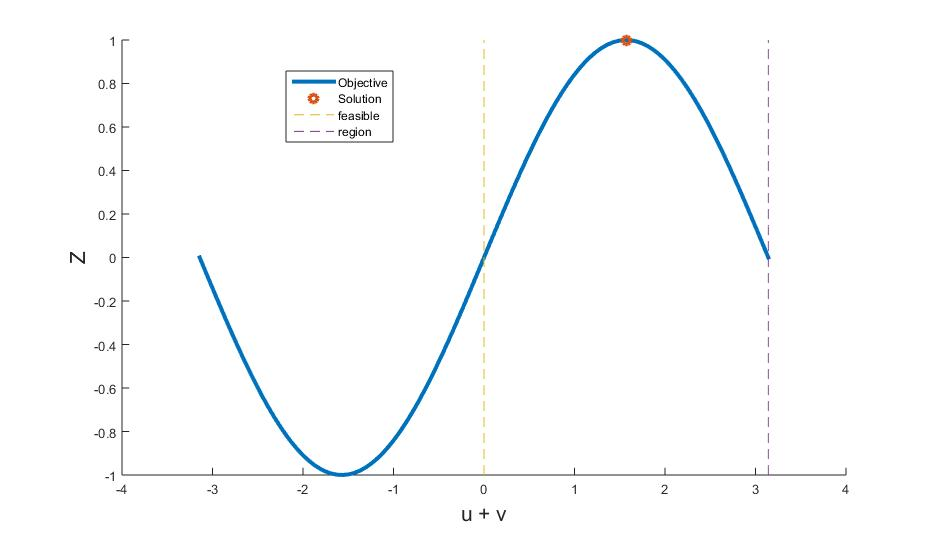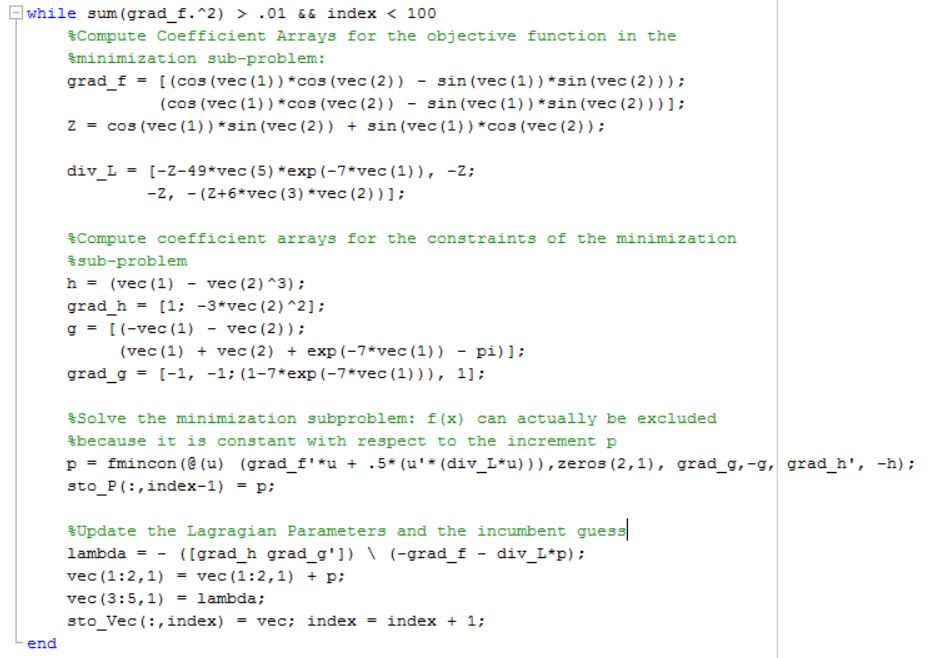# Sequential quadratic programming

(diff) ← Older revision | Latest revision (diff) | Newer revision → (diff)

This web page is a duplicate of https://optimization.mccormick.northwestern.edu/index.php/Sequential_quadratic_programming

Authored by: Ben Goodman (ChE 345 Spring 2016)
Steward: Dajun Yue and Fenqi You

# Introduction

Sequential quadratic programming (SQP) is a class of algorithms for solving non-linear optimization problems (NLP) in the real world. It is powerful enough for real problems because it can handle any degree of non-linearity including non-linearity in the constraints. The main disadvantage is that the method incorporates several derivatives, which likely need to be worked analytically in advance of iterating to a solution, so SQP becomes quite cumbersome for large problems with many variables or constraints. The method dates back to 1963 and was developed and refined in the 1970's . SQP combines two fundamental algorithms for solving non-linear optimization problems: an active set method and Newton’s method, both of which are explained briefly below. Previous exposure to the component methods as well as to Lagrangian multipliers and Karush-Kuhn-Tucker (KKT) conditions is helpful in understanding SQP. The abstracted, general problem below will be used for the remainder of this page to explain and discuss SQP:
${\text{min f(x)}}$${\text{s.t. h(x) = 0}}$${\text{and g(x)}}\leq 0$with f(x), h(x), and g(x) each potentially non-linear. $x$is potentially a vector of many variables for the optimization, in which case h(x) and g(x) are systems.

# Background: Prerequisite Methods

## Karush-Kuhn-Tucker (KKT) Conditions and the Lagrangian Function

The Lagrangian function combines all the information about the problem into one function using Lagrangian multipliers $\lambda$for equality constraints and $\mu$for inequality constraints: $L(x,\lambda ,\mu )$${\text{ = f(x) +}}$$\sum _{i}$$\lambda _{i}h_{i}(x)+$$\sum _{i}$$\mu _{i}g_{i}(x)$A single function can be optimized by finding critical points where the gradient is zero. This procedure now includes $\lambda$and $\mu$as variables (which are vectors for multi-constraint NLP). The system formed from this gradient is given the label KKT conditions:

$\nabla L=$${\begin{bmatrix}{\frac {dL}{dx}}\\{\frac {dL}{d\lambda }}\\{\frac {dL}{d\mu }}\end{bmatrix}}=$${\begin{bmatrix}\nabla f+\lambda \nabla h+\mu \nabla g^{*}\\h\\g^{*}\end{bmatrix}}$$=0$The second KKT condition is merely feasibility; h(x) were constrained to zero in the original NLP. The third KKT condition is a bit trickier in that only the set of active inequality constraints need satisfy this equality, the active set being denoted by $g^{*}$. Inequality constraints that are nowhere near the optimal solution are inconsequential, but constraints that actively participate in determining the optimal solution will be at their limit of zero, and thus the third KKT condition holds. Ultimately, the Lagrangian multipliers describe the change in the objective function with respect to a change in a constraint, so $\mu$is zero for inactive constraints, so those inactive constraints can be considered removed from the Lagrangian function before the gradient is even taken.

## The Active Set Method and its Limitations

The active set method solves the KKT conditions using guess and check to find critical points. Guessing that every inequality constraints is inactive is conventionally the first step. After solving the remaining system for $x$, feasibility can be checked. If any constraints are violated, they should be considered active in the next iteration, and if any multipliers are found to be negative, their constraints should be considered inactive in the next iteration. Efficient convergence and potentially large systems of equations are of some concern, but the main limitation of the active set method is that many of the derivative expressions in the KKT conditions could still be highly non-linear and thus difficult to solve. Indeed, only quadratic problems seem reasonable to tackle with the active set method because the KKT conditions are linear. Sequential Quadratic Programming addresses this key limitation by incorporating a means of handling highly non-linear functions: Newton's Method.

## Newton's Method

The main idea behind Newton's Method is to improve a guess in proportion to how quickly the function is changing at the guess and inversely proportional to how the function is accelerating at the guess. Walking through a few extreme scenarios makes this approach more intuitive: a long, steep incline in a function will not be close to a critical point, so the improvement should be large, and a shallow incline that is rapidly expiring is likely to be near a critical point, so the improvement should be small. The iterations converge to critical values of any function $f$with improvement steps that follow the form below:
$x_{k+1}=$$x_{k}-$${\frac {\nabla f}{\nabla ^{2}f}}$The negative sign is important. Near minimums, a positive gradient should decrease the guess and vice versa, and the divergence is positive. Near maximums, a positive gradient should increase the guess and vice versa, but the divergence is negative. This sign convention also prevents the algorithm from escaping a single convex or concave region; the improvement will reverse direction if it overshoots. This is an important consideration in non-convex problems with multiple local maximums and minimums. Newton's method will find the critical point closest to the original guess. Incorporating Newton's Method into the active set method will transform the iteration above into a matrix equation.

# The SQP Algorithm

Critical points of the objective function will also be critical points of the Lagrangian function and vice versa because the Lagrangian function is equal to the objective function at a KKT point; all constraints are either equal to zero or inactive. The algorithm is thus simply iterating Newton's method to find critical points of the Lagrangian function. Since the Lagrangian multipliers are additional variables, the iteration forms a system:
${\begin{bmatrix}x_{k+1}\\\lambda _{k+1}\\\mu _{k+1}\end{bmatrix}}=$${\begin{bmatrix}x_{k}\\\lambda _{k}\\\mu _{k}\end{bmatrix}}-$$(\nabla ^{2}L_{k})^{-1}\nabla L_{k}$Recall: $\nabla L=$${\begin{bmatrix}{\frac {dL}{dx}}\\{\frac {dL}{d\lambda }}\\{\frac {dL}{d\mu }}\end{bmatrix}}=$${\begin{bmatrix}\nabla f+\lambda \nabla h+\mu \nabla g^{*}\\h\\g^{*}\end{bmatrix}}$Then $\nabla ^{2}L=$${\begin{bmatrix}\nabla _{xx}^{2}L&\nabla h&\nabla g\\\nabla h&0&0\\\nabla g&0&0\end{bmatrix}}$Unlike the active set method, the need to ever solve a system of non-linear equations has been entirely eliminated in SQP, no matter how non-linear the objective and constraints. Theoretically, If the derivative expressions above can be formulated analytically then coded, software could iterate very quickly because the system doesn't change. In practice, however, it is likely that the divergence will not be an invertible matrix because variables are likely to be linearly bound from above and below. The improvement direction "p" for the Newton's Method iterations is thus typically found in a more indirect fashion: with a quadratic minimization sub-problem that is solved using quadratic algorithms. The subproblem is derived as follows:

$p={\frac {\nabla L}{\nabla ^{2}L}}={\frac {(\nabla L)p}{(\nabla ^{2}L)p}}$Since p is an incremental change to the objective function, this equation then resembles a two-term Taylor Series for the derivative of the objective function, which shows that a Taylor expansion with the increment p as a variable is equivalent to a Newton iteration. Decomposing the different equations within this system and cutting the second order term in half to match Taylor Series concepts, a minimization sub-problem can be obtained. This problem is quadratic and thus must be solved with non-linear methods, which once again introduces the need to solve a non-linear problem into the algorithm, but this predictable sub-problem with one variable is much easier to tackle than the parent problem.
${\text{min(p) }}f_{k}(x)+\nabla f_{k}^{T}p+{\frac {1}{2}}p^{T}\nabla _{xx}^{2}L_{k}p$${\text{s.t. }}\nabla h_{k}p+h_{k}=0$${\text{ and }}\nabla g_{k}p+g_{k}=0$## Example ProblemFigure 1: Solution of Example Problem by Inspection.10

${\text{ Max Z = sin(u)cos(v) + cos(u)sin(v)}}$${\text{ s.t. }}0\leq {\text{(u+v)}}\leq \pi$${\text{ }}u=v^{3}$This example problem was chosen for being highly non linear but also easy to solve by inspection as a reference. The objective function Z is a trigonometric identity:
${\text{ sin(u)cos(v) + cos(u)sin(v) = sin(u+v)}}$The first constraint then just restricts the feasible zone to the first half of a period of the sine function, making the problem convex. The maximum of the sine function within this region occurs at ${\frac {\pi }{2}}$, as shown in Figure 1. The last constraint then makes the problem easy to solve algebraically:
$u+v={\frac {\pi }{2}}$$u=v^{3}$$v^{3}+v={\frac {\pi }{2}}$$v=0.883$and $u=v^{3}=0.688$Now, the problem will be solved using the sequential quadratic programming algorithm. The Lagrangian funtion with its gradient and divergence are as follows:
$L=sin(u)cos(v)+cos(u)sin(v)+\lambda _{1}(u-v^{3})+\mu _{1}(-u-v)+\mu _{2}(u+v-\pi )$$\nabla L=$${\begin{bmatrix}{\frac {dL}{du}}\\{\frac {dL}{dv}}\\{\frac {dL}{d\lambda _{1}}}\\{\frac {dL}{d\mu _{1}}}\\{\frac {dL}{d\mu _{2}}}\end{bmatrix}}=$${\begin{bmatrix}cos(v)cos(u)-sin(u)sin(v)+\lambda _{1}-\mu _{1}+\mu _{2}\\cos(v)cos(u)-sin(u)sin(v)-3\lambda _{1}v^{2}-\mu _{1}+\mu _{2}\\u-v^{3}\\-u-v\\u+v-\pi \end{bmatrix}}$$\nabla ^{2}L=$${\begin{bmatrix}-Z&-Z&1&-1&1\\-Z&-(Z+6\lambda _{1}v)&-3v^{2}&-1&1\\1&-3v^{2}&0&0&0\\-1&-1&0&0&0\\1&1&0&0&0\end{bmatrix}}$with $Z=sin(u)cos(v)+cos(u)sin(v)$Figure 2: MATLAB program for performing sequential Newton steps on quadratic subproblem.10

The first important limitation in using SQP is now apparent: the divergence matrix is not invertible because it is not full rank. We will switch to the quadratic minimization sub-problem above, but even with this alternate framework, the gradient of the constraints must be full rank. This can be handled for now by artificially constraining the problem a bit further so that the derivatives of the inequality constraints are not linearly dependent. This can be accomplished through a small modification to the $\mu _{2}$constraint.
If $u+v\leq \pi$, then it is also true that $u+v+exp(-7u)\leq \pi$The addition to the left-hand-side is relatively close to zero for the range of possible values of $u$, so the feasible region has not changed much. This complication is certainly annoying, however, for a problem that's easily solved by inspection. It illustrates that SQP is truly best for problems with highly non-linear objectives and constraints. Now, the problem is ready to be solved. The MATLAB code in figure two was implemented, using the function fmincon to solve the minimization subproblems. fmincon is itself an SQP piece of software. In each step, the incumbent guess is plugged into the gradient, hessian, and constraint arrays, which then become parameters for the minimization problem.

# Conclusion

SQP is powerful enough to be used in commercial software but also burdened by some intricacy. In addition to the complication from needing full-rank constraint gradients, the divergence matrix can be very difficult or laborious to assemble analytically. Commercial SQP packages include checks for the feasibility of the sub-problem in order to account for rank deficiencies. In addition to fmincon, SNOPT and FILTERSQP are two other commercial SQP packages, and each uses a different non-linear method to solve the quadratic subproblem. Line search methods and trust-region methods are trusted options for this step, and sub-gradient methods have also been proposed. The other common modification to SQP (ubiquitous to commercial packages) is to invoke quasi-Newton methods in order to avoid computing the Hessian entirely. SQP is thus very much a family of algorithms rather than a stand-alone tool for optimization. At its core, it is a method for turning large, very non-linear problems into a sequence of small quadratic problems to reduce the computational expense of the problem.

# Sources

 Nocedal, J. and Wright, S. Numerical Optimization, 2nd. ed., Ch. 18. Springer, 2006.
 You, Fengqi. Lecture Notes, Chemical Engineering 345 Optimization. Northwestern University, 2015.